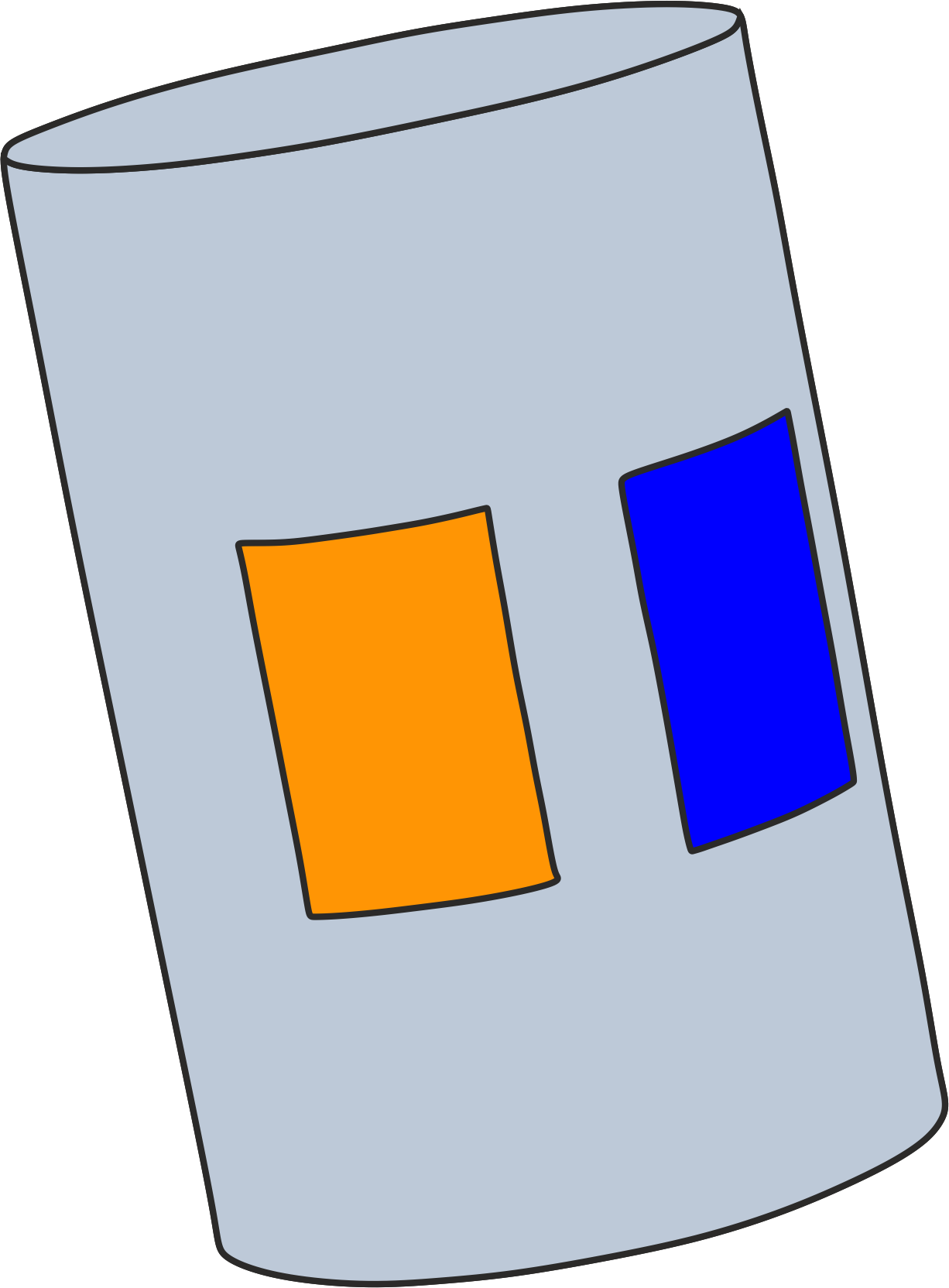# Solve ∇⋅σ∇ϕ=0 in a cylinder...

I want to solve ∇⋅σ∇ϕ=0 in a cylinder with a specific σ. The following figure shows my geometry. I want to set the voltage on my orange electrode to "sin(2pif1t)" and on my blue electrode to "sin(2pif2t)" whwre f1=2000Hz and f2=2050Hz and compute the spatial electric field and potential distribution in my cylinder in time domain. How can I simulate this problem? Is it possible? I investigated EM FDTD, in this solver I cannot apply constant voltage on my electrodes. In ohmic-quasi-static solver, I cannot apply different freauency to different electrodes and extract voltage in time.perhaps use the superposition principle: perform 2 quasi-static simulations where you set the voltage of one electrode to the desired value/frequency and the voltage of the other one to zero. Then you can combine the results using time-dependent weights to get the fields at a specific time point.

@sylvain I learned your before reply.I want to know how to set the voltage of electrode to the desired value or frequency.how to set the expression(sin(2pi*2000t)) in siM4life?
best wishes